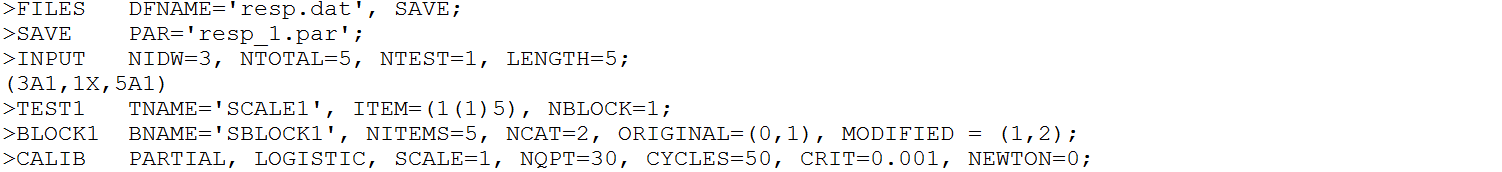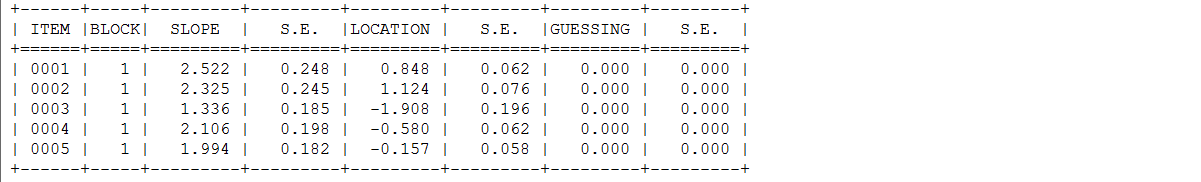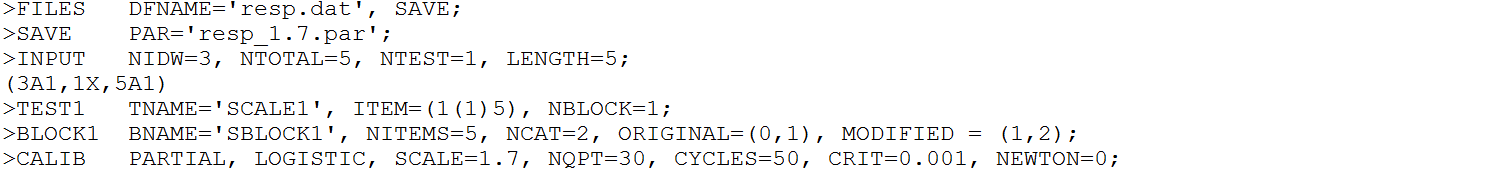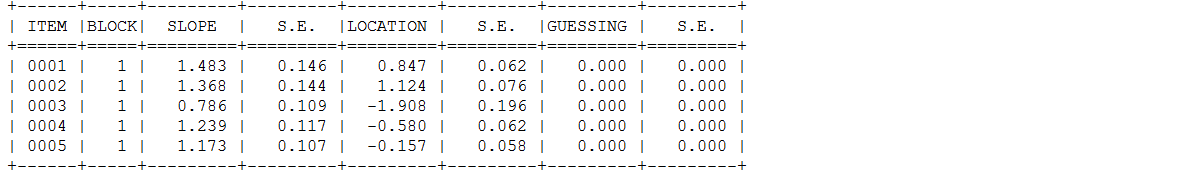Item Response Theory is a comprehensive statistical framework that is used widely in educational and psychological research to model an examinee’s individual response patterns on a test or other instrument by specifying an interaction between the underlying latent trait and item characteristics.

A number of commercial software packages are available for the estimation of IRT models, such as Bilog-MG (Zimowski, Muraki, Mislevy, & Bock, 2006), Multilog (Thissen, 1991), Parscale (Muraki & Bock, 1997), ConQuest (Adams, Wu, & Wilson, 2012), IRTPRO (Cai, du Toit, & Thissen, 2011), and FlexMIRT (Cai, 2012). In recent years, some free IRT packages have been developed in the R environment (R Development Core Team, 2018), such as ltm (Rizopoulos, 2006), mirt (Chalmers, 2012), TAM (Robitzsch, Kiefer, & Wu, 2019), and sirt (Robitzsch, 2019). Many of these tools use different parameterizations of the model, making direct comparisons of results a challenge.

In this blog, we first demonstrate how to obtain comparable item parameter estimates in PARSCALE, mirt, TAM, for the two-parameter IRT model. Second, we demonstrate how to specify item parameters in order to generate response data in lsasim (Matta, Rutkowski, Rutkowski, & Liaw, 2018).

In general, the logistic form of the two-parameter IRT model can be written as

$p(y_{ij} = 1 | \theta_{j}) = \frac{1} {1 + \text{exp} \left[ - Da_{i} (\theta_{j} - b_{i}) \right]}$

where $$y_{ij}$$ is the response to item $$i$$ by respondent $$j$$, $$\theta_{j}$$ is the latent trait for respondent $$j$$, $$D$$ is a scaling constand ($$D$$ = 1.7 to scale the logistic to the normal ogive metric; $$D$$ = 1 to preserve the logistic metric), and $$b_{i}$$ and $$a_{i}$$ are the difficulty parameter and discrimination (slope) parameter, respectively, for item $$i$$.

When models are estimated in the logistic metric, which means that there is no $$D$$ = 1.7 scaling factor, $$a_{i}$$ discrimination (slope) parameters will be approximately 1.7 times higher than they would be if reported in the normal ogive metric.

Install R pacakges.

library(mirt)
library(TAM)
library(lsasim)
packageVersion("mirt")
 '1.30'
packageVersion("TAM")
 '3.2.24'
packageVersion("lsasim")
 '2.0.0.9016'

resp <- read.csv2("C:\\resp.csv", header = F)
colnames(resp) <- c("id", "V1", "V2", "V3", "V4", "V5")
head(resp)
  id V1 V2 V3 V4 V5
1  1  0  0  0  0  0
2  2  0  1  1  1  1
3  3  1  1  1  1  1
4  4  0  0  0  0  0
5  5  0  0  1  1  0
6  6  1  0  1  1  1

### The PARSCALE version

In the PARSCALE parameterization, $$D$$ can be set to either 1 or 1.7.

In the first command file, the scale constant is set to 1.The output reported item parameters estimation in Phase 2, where $$D$$ = 1.In the second command file, the scale constant is set to 1.7 for slope parameters.The output reported item parameters estimation in Phase 2, where $$D$$ = 1.7.When models are estimated in the logistic metric ($$D$$ = 1), discrimination parameters are approximately 1.7 times higher than they reported in the normal ogive metric ($$D$$ = 1.7).

slope_D1_logistic <- c(2.522, 2.325, 1.336, 2.106, 1.994)
slope_D1.7_normal <- c(1.483, 1.368, 0.786, 1.239, 1.173)
slope_D1_logistic/slope_D1.7_normal
 1.700607 1.699561 1.699746 1.699758 1.699915

### The mirt version

In the mirt parameterization, the functions are written with the logistic metric, i.e., $$a_{i}\theta_{j} + d_{i}$$, where $$d_{i}$$ denotes item easiness. For the unidimensional models, the $$d$$ parameters can be converted into traditional IRT $$b$$ parameters. When IRTpars = TRUE, $$b = -d/a$$ while the $$a$$ parameters will be identical under this parameterization.

mmirt <- mirt::mirt(resp[, paste0("V", 1:5)], 1, itemtype = "2PL", verbose = FALSE)
mmirt_coef1 <- mirt::coef(mmirt, simplify = TRUE, IRTpars = FALSE)
mmirt_coef1$items  a1 d g u V1 2.522040 -2.1444262 0 1 V2 2.325332 -2.6195831 0 1 V3 1.335560 2.5464438 0 1 V4 2.103789 1.2154576 0 1 V5 1.991937 0.3083698 0 1 mmirt_coef2 <- mirt::coef(mmirt, simplify = TRUE, IRTpars = TRUE) mmirt_coef2$items
          a          b g u
V1 2.522040  0.8502744 0 1
V2 2.325332  1.1265417 0 1
V3 1.335560 -1.9066488 0 1
V4 2.103789 -0.5777469 0 1
V5 1.991937 -0.1548090 0 1

### The TAM version

In the TAM parameterization, the functions are written with the logistic metric in mind, i.e., $$B_{i} \theta_{j} - xsi_{i}$$, where $$B$$ represents item slopes and $$xsi$$ denotes item difficulties.

mtam <- TAM::tam.mml.2pl(resp = resp[, paste0("V", 1:5)], irtmodel = "2PL", verbose = FALSE)

The first column shows $$B$$ item slopes and the second column shows $$xsi$$ item difficulties. $$B$$ are equivalent to traditional IRT $$a$$ parameters.

cbind(mtam$B[1:5, 2, 1], mtam$xsi[, 1])
       [,1]       [,2]
V1 2.523893  2.1453010
V2 2.323765  2.6181900
V3 1.335626 -2.5465196
V4 2.104199 -1.2156551
V5 1.991855 -0.3084357

In order to get traditional IRT $$b$$ parameters, $$xsi$$ has to be divided by $$B$$.

cbind(mtam$B[1:5, 2, 1], mtam$xsi[, 1]/mtam$B[1:5, 2, 1])  [,1] [,2] V1 2.523893 0.8499967 V2 2.323765 1.1267017 V3 1.335626 -1.9066110 V4 2.104199 -0.5777281 V5 1.991855 -0.1548485 ### The lsasim version The functions of cognitive item responses generation are written with the logistic metric in the lsasim. $$a_{i}$$ and $$b_{i}$$ parameters in the traditional IRT metric are required when users want to specify item parameters. Specify the number of subjects, the number of items, and the number of booklets. N <- 1000 I <- 5 K <- 1 Generate latent trait. theta <- rnorm(N, 0, 1) Specify item parameters. item_pool <- data.frame(item = 1:I, b = c(0.85, 1.13, -1.91, -0.58, -0.15), a = c(2.52, 2.32, 1.34, 2.1, 1.99), c = 0, k = 1, p = 2) Specify rotated booklet design. block_bk1 <- lsasim::block_design(n_blocks = K, item_parameters = item_pool) book_bk1 <- lsasim::booklet_design(item_block_assignment = block_bk1$block_assignment, book_design = matrix(K))

book_samp <- lsasim::booklet_sample(n_subj = N, book_item_design = book_bk1, book_prob = NULL)

Generate cognitive item response data.

cog <- lsasim::response_gen(subject = book_samp$subject, item = book_samp$item, theta = theta,
b_par = item_pool$b, a_par = item_pool$a)
head(cog)
  i001 i002 i003 i004 i005 subject
1    0    0    0    0    0       1
2    1    0    1    1    1       2
3    0    0    1    1    0       3
4    0    0    1    1    1       4
5    0    0    1    1    0       5
6    1    0    1    1    1       6